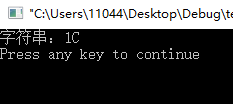2009-07-22 21:17:00 chocolate001 阅读数 11953
• ###### 《C语言基础》 之 第9章 字符串

课程内容：字符数组1、字符串越位的问题、指针变量的空间大小、字符串常量、字符串传参、printf格式化输出字符串、字符串的非格式化输出函数、字符串输入函数、strlen函数、strcpy函数和strncpy函数、strcmp函数和strncmp函数、strcat函数和strncat函数。

3524 人正在学习 去看看 张先凤

C语言提供了几个标准库函数，可以将任意类型(整型、长整型、浮点型等)的数字转换为字 符串，下面列举了各函数的方法及其说明。

itoa()：将整型值转换为字符串。

ltoa()：将长整型值转换为字符串。

ultoa()：将无符号长整型值转换为字符串。

gcvt()：将浮点型数转换为字符串，取四舍五入。

ecvt()：将双精度浮点型值转换为字符串，转换结果中不包含十进制小数点。

fcvt()：指定位数为转换精度，其余同ecvt()

str_int: 435
str_double: 435.10001

itoa()的参数10表示按十进制类型进行转换，转换后的结果是"435"，如果按二进制类型进行转换，则结果为"1101110011"

gcvt()中的参数8表示精确位数，这里得到的结果是"435.10001"

2005-04-23 10:24:00 jammyxu 阅读数 7148
• ###### 《C语言基础》 之 第9章 字符串

课程内容：字符数组1、字符串越位的问题、指针变量的空间大小、字符串常量、字符串传参、printf格式化输出字符串、字符串的非格式化输出函数、字符串输入函数、strlen函数、strcpy函数和strncpy函数、strcmp函数和strncmp函数、strcat函数和strncat函数。

3524 人正在学习 去看看 张先凤

typedef unsigned char BYTE;
BYTE * ByteToStr(BYTE n)
{
BYTE str;
BYTE * pStr;
pStr = str;
if (n>99)
{
*pStr = n/100;
n %= 100;
*pStr += '0';
pStr ++ ;
*pStr = n/10;
n %= 10;
*pStr += '0';
pStr ++ ;
}
if (n>9)
{
*pStr = n/10;
n %= 10;
*pStr += '0';
pStr ++ ;
}
if (n<10)
{
*pStr = n + '0';
pStr ++;
}
*pStr = 0;
return str;
}

2019-01-12 23:09:29 weixin_44453964 阅读数 1211
• ###### 《C语言基础》 之 第9章 字符串

课程内容：字符数组1、字符串越位的问题、指针变量的空间大小、字符串常量、字符串传参、printf格式化输出字符串、字符串的非格式化输出函数、字符串输入函数、strlen函数、strcpy函数和strncpy函数、strcmp函数和strncmp函数、strcat函数和strncat函数。

3524 人正在学习 去看看 张先凤

1.用sprintf
2.用itoa;
3.用子函数然后调用。

SBUF = num/1000+‘0’;
while(!TI);
TI=0;
SBUF = num%1000/100+‘0’;
while(!TI);
TI=0;
SBUF = num%100/10+‘0’;
while(!TI);
TI=0;
SBUF = num%10+‘0’;
while(!TI);
TI=0;

2019-11-16 10:58:06 weixin_43873989 阅读数 51
• ###### 《C语言基础》 之 第9章 字符串

课程内容：字符数组1、字符串越位的问题、指针变量的空间大小、字符串常量、字符串传参、printf格式化输出字符串、字符串的非格式化输出函数、字符串输入函数、strlen函数、strcpy函数和strncpy函数、strcmp函数和strncmp函数、strcat函数和strncat函数。

3524 人正在学习 去看看 张先凤

## 单片机中十进制转十六进制

``````u8 temp;
u8 tempHexstr;

void nToHexstr(u8 n, u8 * hexstr, u8 strlen)
{
u8 hexChar = {'0', '1','2','3', '4','5','6', '7','8','9', 'A','B','C', 'D','E','F'};
u8 i;
u8 dis;
u32 nTemp = (int32_t) n;
for(i=1; i<=strlen; i++)
{
dis = nTemp & 0x0f;
hexstr[strlen-i] = hexChar[dis];
nTemp = nTemp >>4;
}
hexstr[strlen]='\0';  //使用strcat()函数，进行字符串拼接。需要结束符
}

printf("温度：%c \n",tempHexstr);
``````

``````#include<stdio.h>

void nToHexstr(unsigned char n, unsigned char * hexstr, unsigned char strlen)
{
unsigned char hexChar = {'0', '1','2','3', '4','5','6', '7','8','9', 'A','B','C', 'D','E','F'};
unsigned char i;
unsigned char dis;
unsigned long nTemp = (long) n;
for(i=1; i<=strlen; i++)
{
dis = nTemp & 0x0f;
hexstr[strlen-i] = hexChar[dis];
nTemp = nTemp >>4;
}
hexstr[strlen]='\0';
}

void main()
{
unsigned char temp=28;

unsigned char tempHexstr;

nToHexstr(temp, tempHexstr, 2);//n=28   hex:001C

printf("字符串：%s \n",tempHexstr);

}
``````

28 -->1C2012-12-29 15:24:23 fcf1990501 阅读数 199
• ###### 《C语言基础》 之 第9章 字符串

课程内容：字符数组1、字符串越位的问题、指针变量的空间大小、字符串常量、字符串传参、printf格式化输出字符串、字符串的非格式化输出函数、字符串输入函数、strlen函数、strcpy函数和strncpy函数、strcmp函数和strncmp函数、strcat函数和strncat函数。

3524 人正在学习 去看看 张先凤

 `01` `#include `
 `02` `#include `
 `03` `char` ``` *digitToAlpha (````int` ``` val, ````char` ```*buf, unsigned radix); ```
 `04` `int` ``` main(````int` ```argc, ````char` ```*argv[]) ```
 `05` `{ `
 `06` `  ``int` `iNum = 55; `
 `07` `  ``char` `strNum = ``""````; ```
 `08` `  ````digitToAlpha(iNum,strNum,10); ```
 `09` `  ``printf``(``"%s \n"````,strNum); ```
 `10` `   `
 `11` `  ``system``(``"PAUSE"````);     ```
 `12` `  ``return` `0; `
 `13` `} `
 `14` `/* `
 `15` `功能:将数值转换为字符串 `
 `16` `参数:第一个是要转化的整数    `
 `17` `     ````第二个是转化后的字符串 ```
 `18` `     ````第三个是要转化整数的基数，就是说如果基数是10，就可以直接转化，如果不是10，是其他值（2-36之间），则先把该整数转化为该基数的数后，再转化为字符串 ```
 `19` `*/`
 `20` `char` ``` *digitToAlpha (````int` ``` val, ````char` ```*buf, unsigned radix)  ```
 `21` `{  `
 `22` `    ``char` `*p; ``/* pointer to traverse string */`
 `23` `    ``char` `*firstdig;``/* pointer to first digit */`
 `24` `    ``char` `temp; ``/* temp char */`
 `25` `    ````unsigned digval; ````/* value of digit */`
 `26` `    ````p = buf;  ```
 `27` `    ``if````(val<0) ```
 `28` `    ``{  `
 `29` `        ``/* negative, so output '-' and negate */`
 `30` `        ````*p++= ````'-'``;  `
 `31` `        ````val = (unsigned ````long``)(-(``long````)val);  ```
 `32` `    ``}  `
 `33` `    ``firstdig = p;``/* save pointer to first digit */`
 `34` `    ``do` `{  `
 `35` `        ````digval = (unsigned)(val%radix);  ```
 `36` `        ````val /=radix; ````/* get next digit */`
 `37` `        ``/* convert to ascii and store */`
 `38` `        ``if` `(digval > 9)  `
 `39` `            ``*p++ = (``char````) (digval - 10 + ````'a'``); ``/* a letter */`
 `40` `        ``else`
 `41` `            ``*p++ = (``char````) (digval + ````'0'``); ``/* a digit */`
 `42` `    ``} ` `while``(val > 0);  `
 `43` `    ````/* We now have the digit of the number in the buffer, but in reverse  ```
 `44` `    ``order. Thus we reverse them now. */`
 `45` `    ````*p-- = ````'\0'``; ``/* terminate string; p points to last digit */`
 `46` `    ``do`
 `47` `    ``{  `
 `48` `        ````temp = *p;  ```
 `49` `        ````*p =*firstdig;  ```
 `50` `        ````*firstdig= temp; ````/* swap *p and *firstdig */`
 `51` `        ````--p;  ```
 `52` `        ````++firstdig;     ````/* advance to next two digits */`
 `53` `    ``} ` `while` ```(firstdig < p); ````/* repeat until halfway */`
 `54` `    ``return` `buf;  `
 `55` `}`

http://www.nowamagic.net/librarys/veda/detail/482